##帐号 自动登录 找回密码 密码 立即注册

# Proteus仿真《用51单片机制作的6位数码管GPS授时时钟》[复制链接]ID:149389 发表于 2019-5-18 14:08 | 显示全部楼层 |阅读模式
 原贴: http://www.51hei.com/bbs/dpj-100009-1.html 仿真环境：Proteus 8.5 SP0 仿真说明：在Proteus仿真中使用AT89C52单片机，在原始程序的基础上，调整了部分引脚定义，只测试了GPS授时功能。仿真不包含红外部分。 1、为了对照说明GPS授时效果，DS1302，取消该选项的勾选。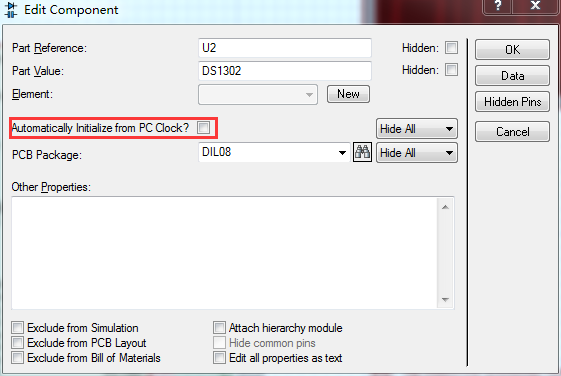2、仿真开始，时间从0:00:00 开始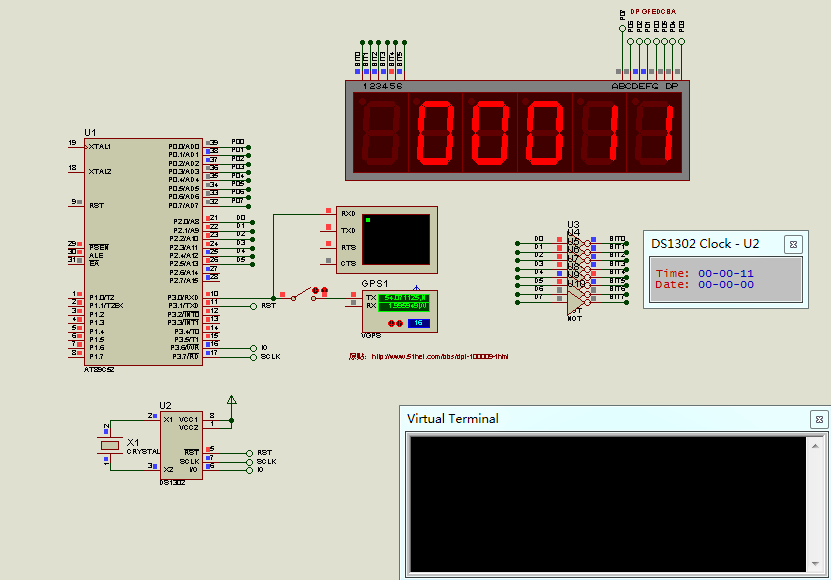3、闭合开关，获取GPS授时信息，GPS授时的标记点亮，串口可观察GPS信息，DS1302成功写入获取的时间。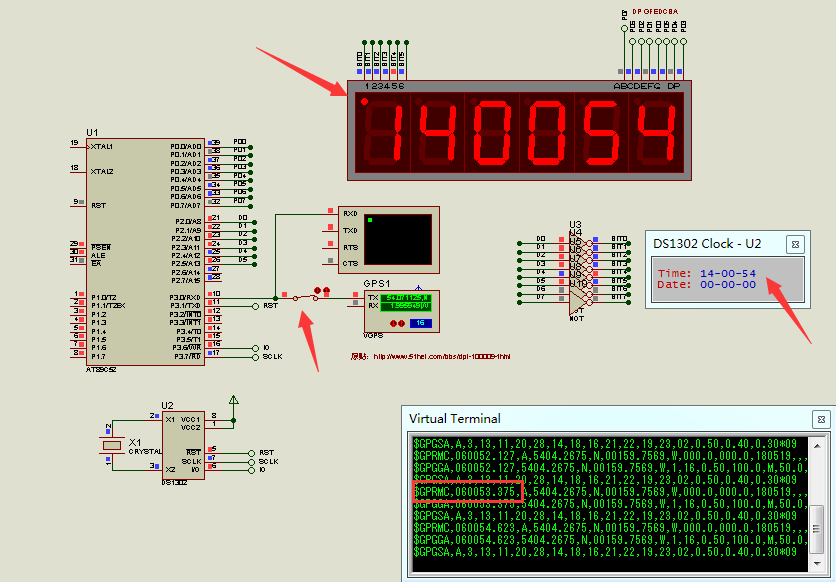4、断开开关，时钟从DS1302获取时间值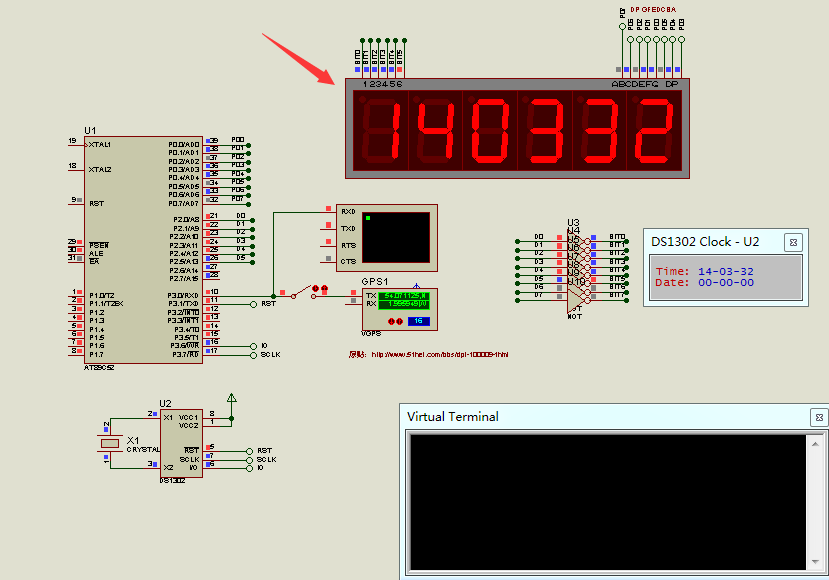5、仿真文件，含Proteus 8.5格式的工程文件，以及Hex文件，程序请参照原贴。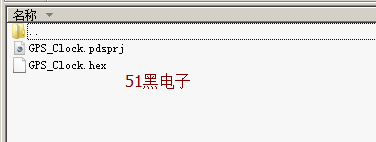全部资料51hei下载地址(仿真+hex文件 不带源码)：6位数码管GPS时钟.rar (20.61 KB, 下载次数: 168) 2019-5-18 14:05 上传 点击文件名下载附件

### 评分ID:601774 发表于 2019-8-23 08:17 | 显示全部楼层
 谢谢，学习了ID:601774 发表于 2019-8-23 08:18 | 显示全部楼层
 谢谢，学习了，刚好准备弄一个，就是不知道gps串口读取的时间精度是到哪一位的，只是到秒级，还是能读取毫秒，微秒ID:99130 发表于 2020-2-17 22:45 来自手机 | 显示全部楼层
 正好需要ID:637324 发表于 2020-3-21 13:21 | 显示全部楼层
 原贴不存在了，想看楼主大哥的程序ID:149389 发表于 2020-4-9 08:58 | 显示全部楼层
 gspt 发表于 2020-3-21 13:21 原贴不存在了，想看楼主大哥的程序 C源程序，main.rar (3.36 KB, 下载次数: 36) 2020-4-9 08:56 上传 点击文件名下载附件 #include //--------数码管段选定义-------- #define LEDPORT P0 #define uchar unsigned char #define uint unsigned int //--------数码管位选定义-------- sbit D1=P2^0; sbit D2=P2^1; sbit D3=P2^2; sbit D4=P2^3; sbit D5=P2^4; sbit D6=P2^5; //--------DS1302接口定义-------- sbit CLK =P3^7;                                          sbit IO  =P3^6; sbit RST =P3^1; //--------状态标记-------- sbit LED1=P2^6; sbit LED2=P2^7; sbit ACC0=ACC^0; sbit ACC7=ACC^7; unsigned char hour,min,sec;                //小时、分钟、秒 bit rev_start,rev_stop;                        //GPS接收开始、结束标志位 unsigned char code tab[ ]={   0xc0,0xf9,0xa4,0xb0,0x99,0x92,0x82,0xf8,0x80,0x90,0xFF};//共阳极0-9,灭 unsigned char  buf;         //GPS数据接收缓冲 unsigned char  irtime;//红外用全局变量,用于计算2个下降沿之间的时间 bit decode_ok,irok; static unsigned char ircode; unsigned char irdata; uchar set,temp,dot; bit flag,test;                                           uint cnt; /****************************************************************************************** *函数名称：Timer_Init *功能描述：定时器初始化 ******************************************************************************************/ void Timer_Init() {   TMOD=0x22;   TH0=0x00; //重载值   TL0=0x00; //初始化值   ET0=1;    //开中断   TR0=1;   IT0 = 1;   //指定外部中断0下降沿触发，INT0 (P3.2)   EX0 = 1;   //使能外部中断   TL2 = 0xCD;                //设置定时初值   TH2 = 0xF8;                //设置定时初值   RCAP2L = 0xCD;        //设置定时重载值   RCAP2H = 0xF8;        //设置定时重载值   ET2=1;   TR2=1;   TH1 = 0xFD;                //9600波特率的初值   TL1 = TH1;                //9600波特率的初值   TR1 = 1;     SCON = 0x50;        //使用串行工作方式1，10位异步收发8位数据，波特率可变（由T1的溢出率控制）   //        ES = 1;   EA=1; } //--------数据串行输入-------- void inputbyte(unsigned char ucDa) {   unsigned char i;   ACC = ucDa;   for(i=8; i>0; i--)   {     IO = ACC0;                   //相当于汇编中的 RRC     CLK = 1;     CLK = 0;     ACC = ACC >> 1;   } } //--------数据并行输出-------- unsigned char outputbyte(void) {   unsigned char i;   for(i=8; i>0; i--)   {     ACC = ACC >>1;                      //相当于汇编中的 RRC     ACC7 = IO;     CLK = 1;     CLK = 0;   }   return(ACC); } //--------数据写入-------- void write(unsigned char ucAddr, unsigned char ucDa) {   RST = 0;   CLK = 0;   RST = 1;   inputbyte(ucAddr);                    //地址，命令   inputbyte(ucDa);                      //写1Byte数据   CLK = 1;   RST =0; } //--------数据读出-------- unsigned char read(unsigned char ucAddr) {   unsigned char ucDa;   RST = 0;   CLK = 0;   RST = 1;   inputbyte(ucAddr);                    //地址，命令   ucDa = outputbyte();                 //读1Byte数据   CLK = 1;   RST =0;   return(ucDa); } //--------DS1302初始化-------- void DS1302_init() {   if(read(0xc1)!=0x82)  //如果1302掉电，写入下面的初始值   {     write(0x8e,0x00);//关闭写保护     write(0x80,0x22); //设置秒     write(0x82,0x22);//设置分钟     write(0x84,0x22); // 小时     write(0x90,0xa5);//涓流充电     write(0xc0,0x82);//掉电标志位   } } //------------------------------------------------------------ //--------------遥控器操作部分-------------------------------- void decode(void)//红外码值处理函数 {   unsigned char i, j, k;   unsigned char cord,value;   k=1;   for(i=0;i<4;i++)      //处理4个字节   {     for ( j=1; j<=8;  j++) //处理1个字节8位     {       cord=irdata[k];       if(cord>7)//大于某值为1，这个和晶振有绝对关系，这里使用12M计算，此值可以有一定误差       {         value=value|0x80;       }       else       {         value=value;       }       if(j<8)       {         value=value>>1;       }       k++;     }     ircode[i]=value;     value=0;        }   if(ircode==~ircode)     decode_ok=1;//解码完毕后标志位置1 } void ir_work() {   if(ircode==0x1e)     test=!test;                //熄灭屏幕标记   if(ircode==0x05)   {     set++;     if(set==4)       set=0;   }   if(set==1)   {     if(ircode==0x02|ircode==0x06)                                                //hour     {       temp=(read(0x85)/16)*10+read(0x85)%16;       temp++;       if(temp==24)temp=0;       write(0x84,(temp/10)*16+temp%10);     }     if(ircode==0x08|ircode==0x04)     {       temp=(read(0x85)/16)*10+read(0x85)%16;       temp--;       if(temp==-1)temp=23;       write(0x84,(temp/10)*16+temp%10);     }            }   if(set==2)                                                                         //min   {     if(ircode==0x02|ircode==0x06)            {       temp=(read(0x83)/16)*10+read(0x83)%16;       temp++;       if(temp==60)temp=0;       write(0x82,(temp/10)*16+temp%10);     }     if(ircode==0x08|ircode==0x04)     {       temp=(read(0x83)/16)*10+read(0x83)%16;       temp--;       if(temp==-1)temp=59;       write(0x82,(temp/10)*16+temp%10);     }            }   if(set==3)                                                                         //sec   {     if(ircode==0x02|ircode==0x06)            {       temp=(read(0x81)/16)*10+read(0x81)%16;       temp++;       if(temp==60)temp=0;       write(0x80,(temp/10)*16+temp%10);     }     if(ircode==0x08|ircode==0x04)     {       temp=(read(0x81)/16)*10+read(0x81)%16;       temp--;       if(temp==-1)temp=59;       write(0x80,(temp/10)*16+temp%10);     }            }   decode_ok=0;        } //--------数码管显示-------- void Display(unsigned char a,b,c)   //数码管显示 {   static unsigned char i;   LEDPORT=0xff;   switch (i)   {   case 0:     if(a/16)                                //不为零则显示       LEDPORT=tab[a/16]˙     else       LEDPORT=tab˙        //最高位"0"，消隐字符     D1=0;     D2=D3=D4=D5=D6=1;            break;   case 1:     LEDPORT=tab [a%16];        D2=0;     D1=D3=D4=D5=D6=1;        break;   case 2:     LEDPORT=tab [b/16];                 D3=0;     D1=D2=D4=D5=D6=1;            break;   case 3:     LEDPORT=tab [b%16];            D4=0;     D1=D2=D3=D5=D6=1;            break;   case 4:     LEDPORT=tab [c/16];                 D5=0;     D1=D2=D3=D4=D6=1;            break;   case 5:     LEDPORT=tab [c%16];        D6=0;     D1=D3=D4=D5=D2=1;            break;   }   i++;   if(i==6)i=0; } /****************************************************************************************** *函数名称：main *功能描述：主函数 ******************************************************************************************/ void main() {   Timer_Init();   DS1302_init();   dot=0xFF;   flag=1;   while(1)   {                      hour= read(0x85);     min = read(0x83);     sec  = read(0x81);     /*        if((hour==0x08)&&(min==0x00) )                         //可以设置为每天的某个时间打开GPS对一下时间再关掉，这里设置的是8：00              ES=1;              else              ES=0;*/     if(flag==1&&cnt>500)                                  //上电稍做延时再打开串口，防止数码管显示出错     {       flag=0;       cnt=0;       ES=1;     }     if((rev_stop==1)&&(buf=='M')&&(buf=='C')&&(buf==','))//如果接收到GPRMC        就把GPS接收到的时间写入DS1302     {       LED1=1;       ES=0;       hour= (buf -0x30)* 16+ buf -0x30;       min=  (buf -0x30)* 16+ buf-0x30;       sec=  (buf-0x30)* 16+ buf-0x30;       hour=  hour / 16 * 10 + hour % 16;       hour= (hour+8) % 24;        //UTC Time换算成北京时间       hour=hour/10*16+hour%10;       write(0x84,hour);       write(0x82,min);       write(0x80,sec);              ES=1;       rev_stop=0;     }                            if( sec==  (buf-0x30)* 16+ buf-0x30)                                        //用左上角的小点显示有无GPS信号       dot=0x7f;     else       dot=0xff;     if(irok)                         //如果接收到正确的红外信号后进行红外处理     {          decode();       irok=0;     }     if(decode_ok)                //如果解码正确就进入调时程序     {       ir_work();     }     if(test)                        //需要时可以遥控关闭屏幕     {       ET2=0;       P0=0xff;       LED1=LED2=1;     }     else     {       ET2=1;     }             }                     } void int0 (void) interrupt 0 //外部中断0服务函数           用于红外解码 {   static unsigned char  i;             //接收红外信号处理   static bit startflag;                //是否开始处理标志位   if(startflag)                           {     if(irtime<63&&irtime>=33)//引导码 TC9012的头码，9ms+4.5ms       i=0;                       irdata[i]=irtime;//存储每个电平的持续时间，用于以后判断是0还是1     irtime=0;     i++;     if(i==33)     {       irok=1;       i=0;     }   }   else   {     irtime=0;     startflag=1;   } } /****************************************************************************************** *函数名称：timer0 *功能描述：红外解码计算脉冲宽度 ******************************************************************************************/ void timer0 (void) interrupt 1 //定时器0中断服务函数 ，红外解码 {   irtime++; //计算2个下降沿之间的时间 } void Uart_Receive(void) interrupt 4         //串口中断，用于GPS数据接收 {   unsigned char ch,num;   ES = 0;   if (RI)                                        //如果接收完成则进入   {     ch = SBUF;     if (ch == 'R')  //如果收到字符' ，便开始接收     {       rev_start = 1;       rev_stop  = 0;                  //接收停止标志     }     if (rev_start == 1)       //标志位为1，开始接收     {       buf[num++] = ch;  //字符存到数组中       if (ch == '.')       //如果接收到换行       {         buf[num] = '\n';         rev_start = 0;         rev_stop  = 1;          //接收停止标志         num = 0;       }     }   }   RI = 0;         //RI清0，重新接收   ES = 1;         //串口1中断允许                        } /****************************************************************************************** *函数名称：Timer2 *功能描述：定时刷新显示 ******************************************************************************************/ void Timer2() interrupt 5                                //2mS                          //用于刷新数码管的显示 {   TF2=0;          //定时器2必须由软件对溢出标志位清零，硬件不能清零，这里与定时器0和定时器1不同!!!   switch(set)   {   case 0:     Display(hour,min,sec);     LED1=0;     LED2=0;     break;   case 1:     Display(hour,0xaa,0xaa);     LED1=0;     LED2=1;     break;   case 2:     Display(0xaa, min,0xaa);     break;   case 3:     Display(0xaa, 0xaa,sec);     LED1=1;     LED2=0;     break;   }   cnt++; } 复制代码ID:637324 发表于 2020-4-27 09:24 | 显示全部楼层
 weidoit 发表于 2020-4-9 08:58 C源程序， 谢谢！ID:762924 发表于 2020-6-1 15:40 | 显示全部楼层
 weidoit 发表于 2020-4-9 08:58 C源程序， 老哥，那个电路图有吗，楼主的文件打不开（闪退）ID:771461 发表于 2020-6-6 21:25 | 显示全部楼层
 很有帮助，很需要这个资料的内容进行学习。ID:653173 发表于 2020-8-13 10:01 | 显示全部楼层
 楼主能否分享一个Proteus8.5能用的key?ID:889564 发表于 2021-3-8 15:17 | 显示全部楼层
 没有VGPS这个元件啊ID:611269 发表于 2021-8-7 21:33 | 显示全部楼层
 非门是干啥的呀，没搞懂ID:51443 发表于 2021-9-8 17:22 | 显示全部楼层
 有个疑问，一旦接收到GPS信号，就把时间写入DS1302中，这样不停的写入，DS1302中储存器的写入次数寿命到底能撑多久？

 本版积分规则 回帖后跳转到最后一页